## 2-1 Ajax 簡介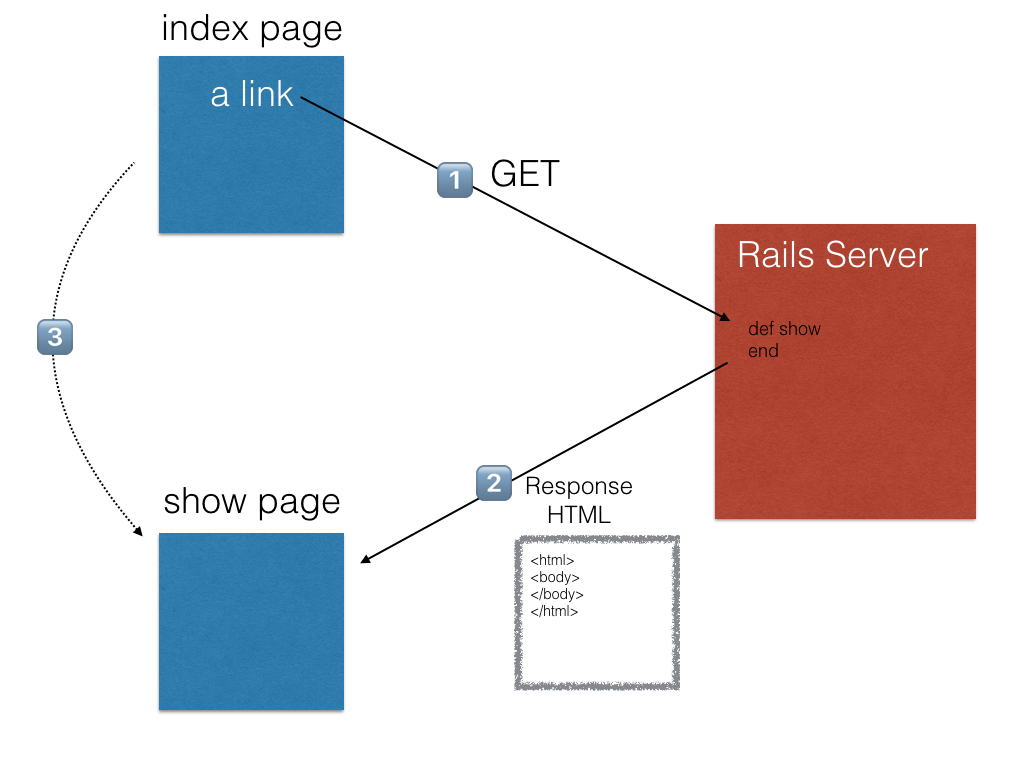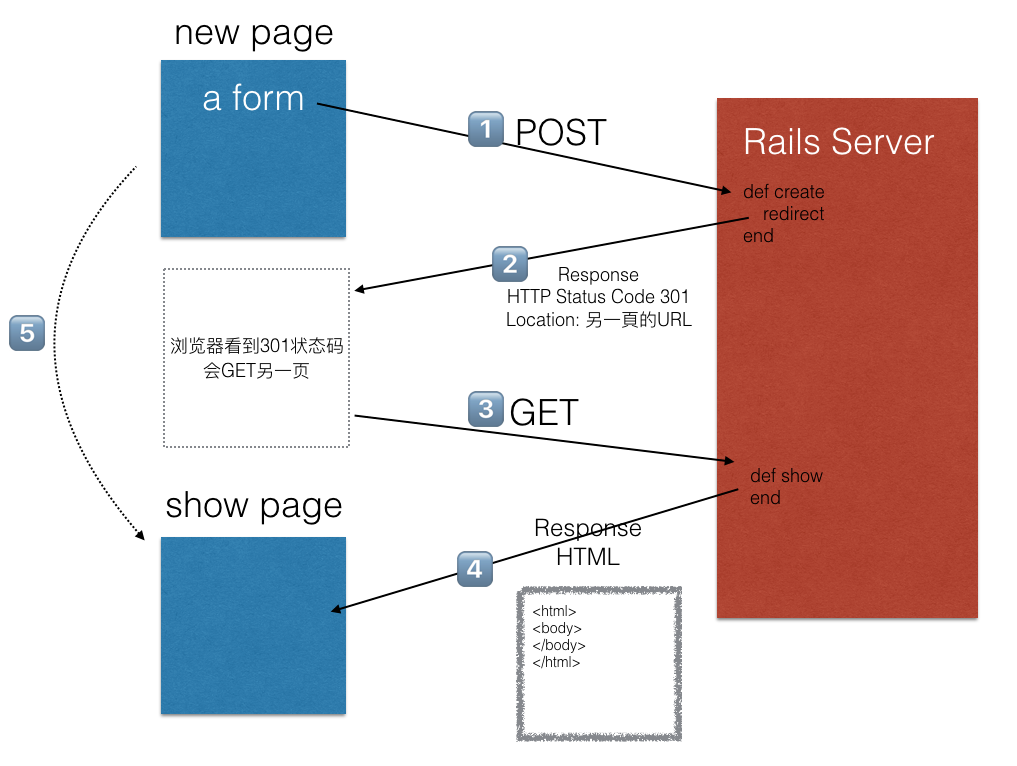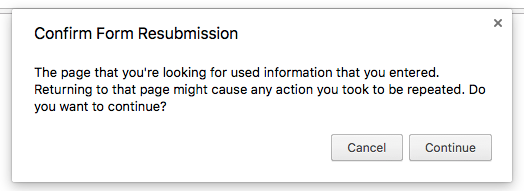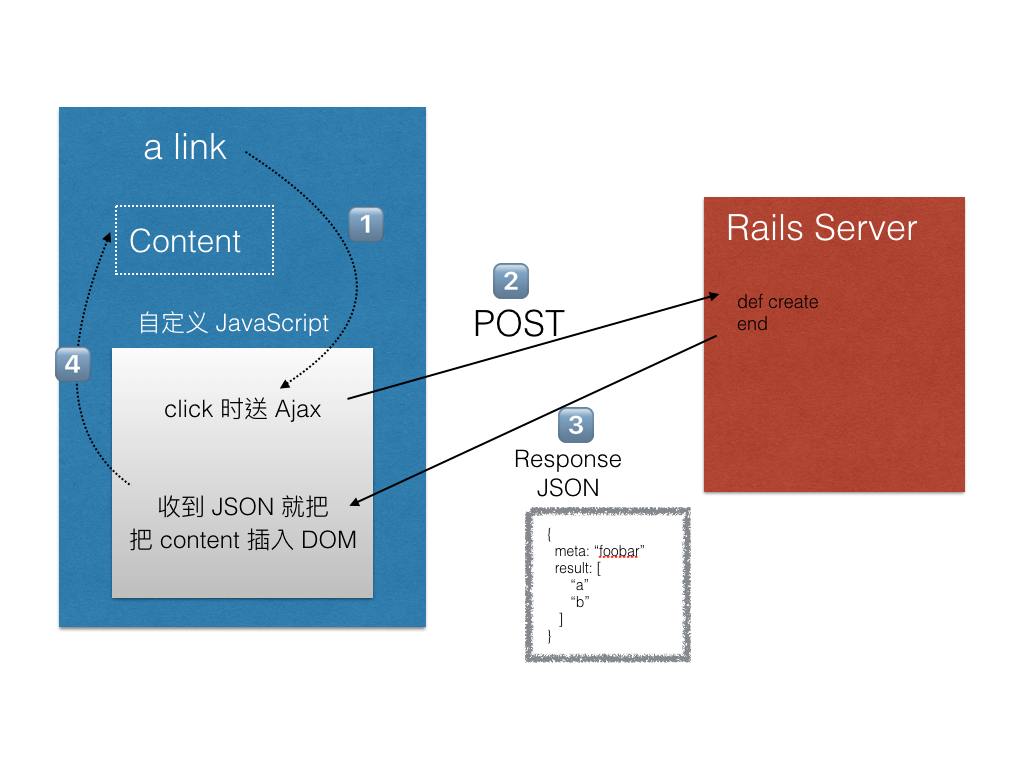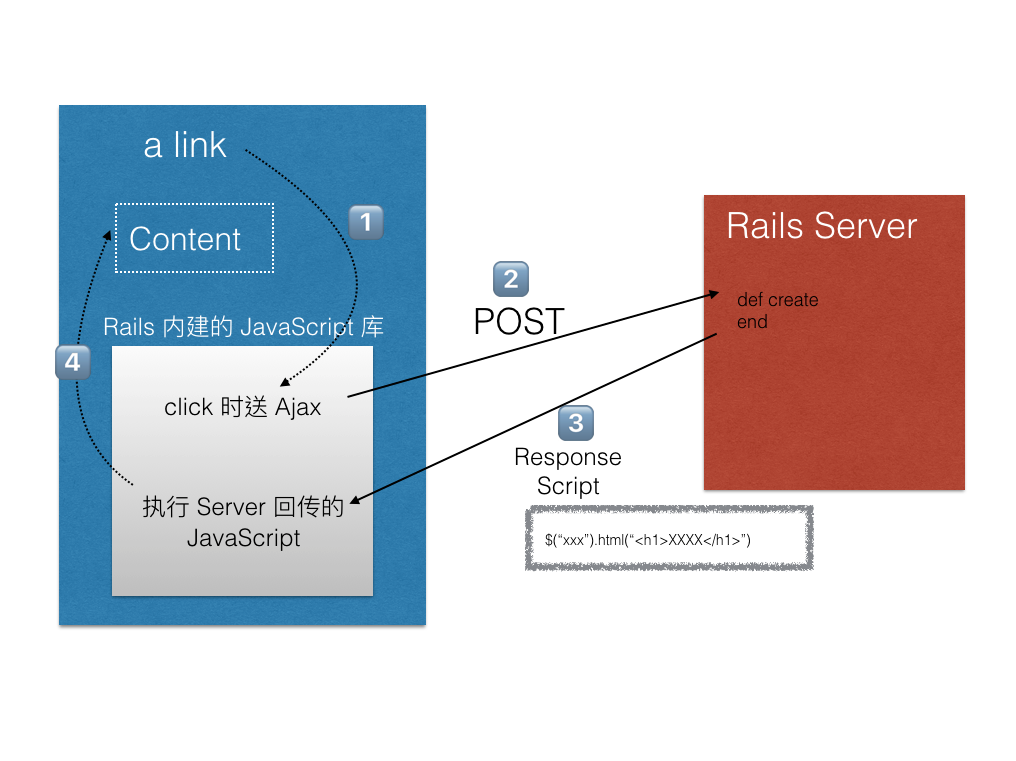## 2-2 目標

• 用戶可以貼文
• 用戶可以瀏覽所有貼文
• 用戶可以刪除自己的貼文
• 用戶可以針對貼文按讚，每篇貼文只能按讚一次

## 2-3 建立 Models

``````  <body>
+   <% if flash[:notice] %>
+     <%= flash[:notice] %>
+   <% end %>

+   <% end %>

+  <% if current_user %>
+     <%= link_to('登出', destroy_user_session_path, :method => :delete) %>
+  <% else %>
+    <%= link_to('註冊', new_registration_path(:user)) %> |
+   <% end %>

...(略)
``````

``````  class User < ApplicationRecord

+   has_many :posts

+   def display_name
+     # # 取 email 的前半來顯示，如果你也可以另開一個字段是 nickname 讓用戶可以自己編輯顯示名稱
+     self.email.split("@").first
+   end

...(略)
``````

``````  class Post < ApplicationRecord
+   validates_presence_of :content
+   belongs_to :user

...(略)
``````

## 2-4 瀏覽貼文和產生假資料

``````
+ resources :posts

- root "pages#jquery_1"
+ root "posts#index"

``````

``````
+  def index
+    @posts = Post.order("id DESC").all    # 新貼文放前面
+  end

``````

``````<% @posts.each do |post| %>

<div class="panel panel-default">
<div class="panel-body">
<%= post.content %>
</div>
</div>

<% end %>

``````

Faker 這個 gem 可以隨機產生假文，編輯 Gemfile 加上：

``````  group :development do
+   gem 'faker'

# (略)
``````

``````
namespace :dev do

users = []
10.times do
users << User.create!( :email => Faker::Internet.email, :password => "12345678")
end

50.times do |i|
post = Post.create!( :content => Faker::Lorem.paragraph,
:user_id => users.sample.id )

end
end

end

``````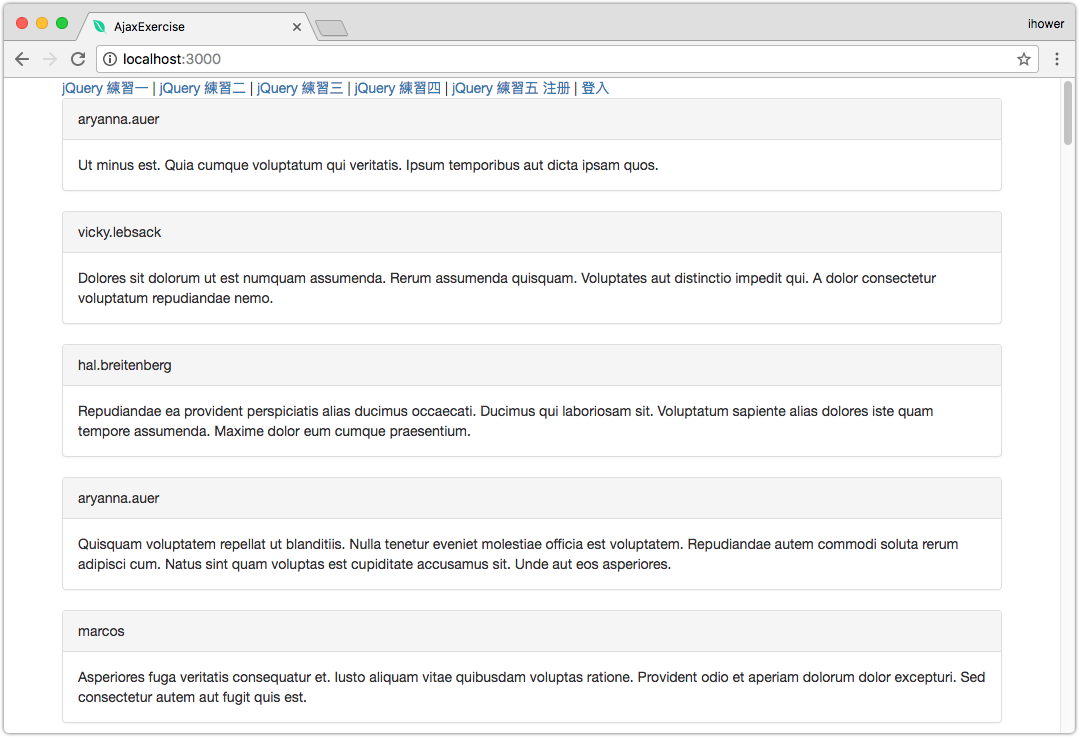## 2-5 新增和刪除貼文

``````  class PostsController < ApplicationController

+   before_action :authenticate_user!, :only => [:create, :destroy]

+   def create
+     @post = Post.new(post_params)
+     @post.user = current_user
+     @post.save
+
+     redirect_to posts_path
+   end
+
+   def destroy
+     @post = current_user.posts.find(params[:id]) # 只能刪除自己的貼文
+     @post.destroy
+
+     redirect_to posts_path
+   end
+
+   protected
+
+   def post_params
+     params.require(:post).permit(:content)
+    end

end

``````

``````+ <%= form_for Post.new do |f| %>
+   <div class="form-group">
+     <%= f.text_area :content, :class => "form-control" %>
+   </div>
+   <div class="form-group">
+     <%= f.submit :class => "btn btn-primary" %>
+   </div>
+ <% end %>

<% @posts.each do |post| %>

<div class="panel panel-default">
<div class="panel-body">
<%= post.content %>

+     <% if current_user && post.user == current_user %>
+       <p class="text-right">
+         <%= link_to "Delete", post_path(post), :method => :delete, :class => "btn btn-danger" %>
+       </p>
+     <% end %>
</div>
</div>

<% end %>
``````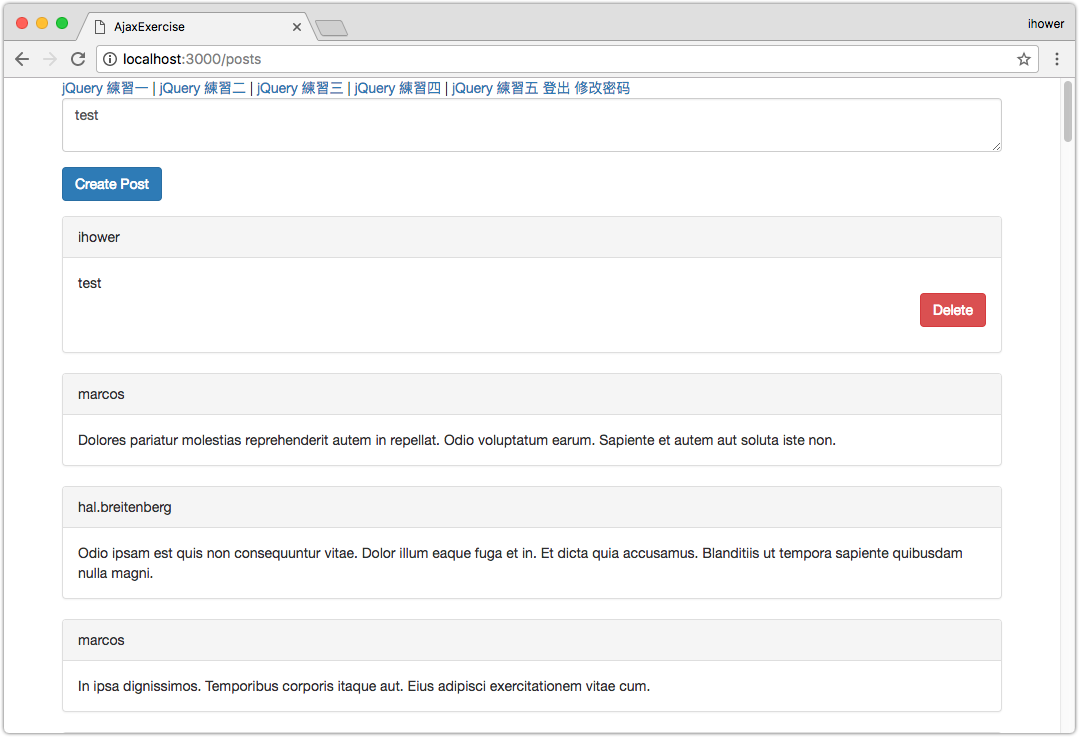## 2-6 Ajax 刪除

``````-  <%= link_to "Delete", post_path(post), :method => :delete, :class => "btn btn-danger" %>
+  <%= link_to "Delete", post_path(post), :method => :delete, :remote => true, :class => "btn btn-danger" %>
``````

``````
def destroy
@post = current_user.posts.find(params[:id])
@post.destroy

-   redirect_to posts_path
end

``````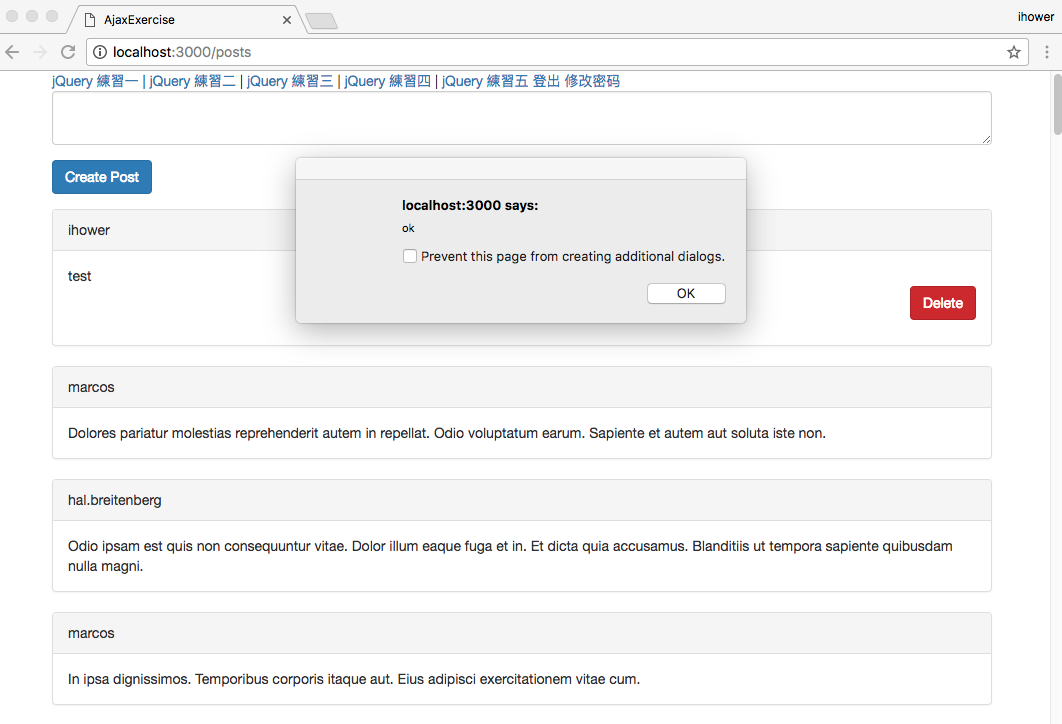``````
def destroy
@post = current_user.posts.find(params[:id])
@post.destroy

end

``````

``````-  <div class="panel panel-default">
+  <div id="post-<%= post.id %>" class="panel panel-default">
``````

``````  \$("#post-<%= @post.id %>").remove();

``````

• 針對要被移除的 `<div>` 區塊，我們補上了一個 id，例如 `<div id="post-123">`，這樣等會 jQuery 要移除的時候，就可以直接抓到要移除哪一個元素了
• 一個 action 如果沒有寫明 `redirect``render` 的話，就會預設去找 action 名稱的樣板。於是這裡就會去找 `destroy.js.erb`
• `destroy.js.erb` 裡面要寫回傳的 JavaScript 代碼，這個檔案也是 erb 樣板，所以可以用 `<%= XXX %>` 內嵌 Ruby 語法

### Ajax 要如何除錯?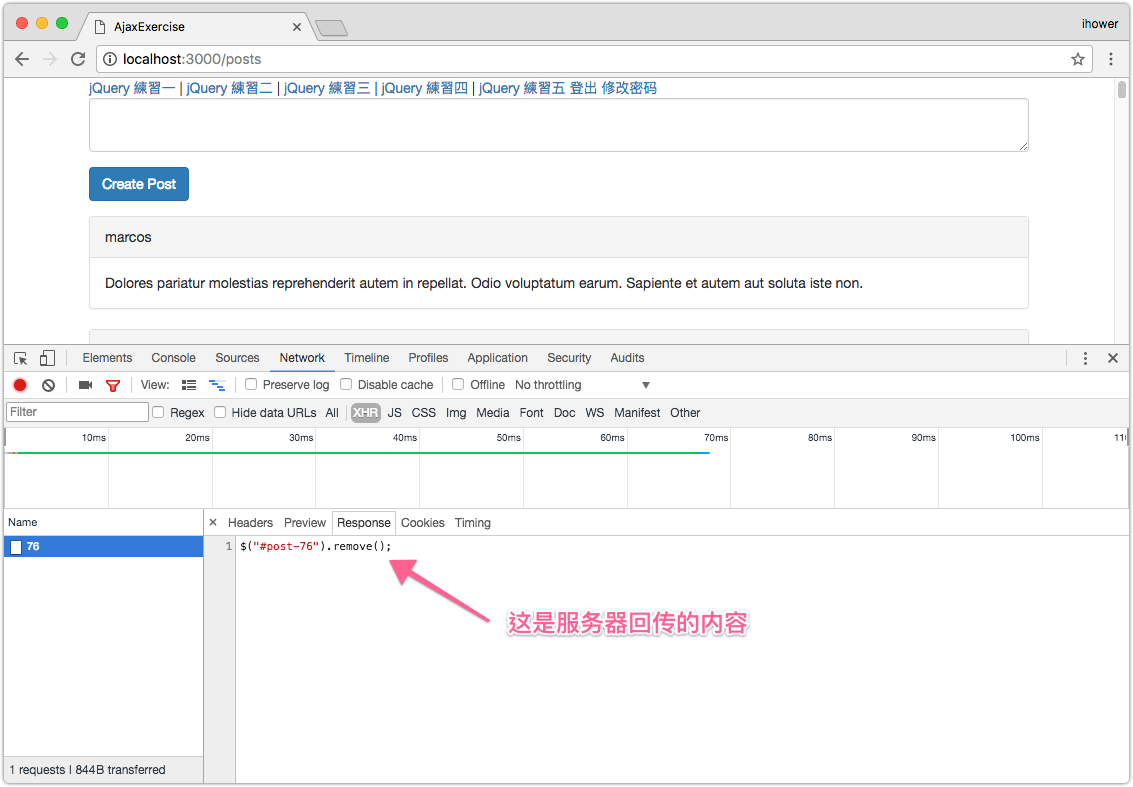## 2-7 Ajax 新增

``````-  <%= form_for Post.new do |f| %>
+  <%= form_for Post.new, :remote => true do |f| %>

``````

``````
def create
@post = Post.new(post_params)
@post.user = current_user
@post.save

-   redirect_to posts_path

end
``````

``````+  <div id="post-list">
<% @posts.each do |post| %>
# 略...
<% end %>
+ </div>

``````

``````  <div id="post-list">
<% @posts.each do |post| %>
-    <div id="post-<%= post.id %>" class="panel panel-default">
-      <%= post.user.display_name %>
-    </div>
-    <div class="panel-body">
-      <%= post.content %>
-
-      <% if current_user && post.user == current_user %>
-        <p class="text-right">
-          <%= link_to "Delete", post_path(post), :method => :delete, :class => "btn btn-danger", :remote => true %>
-        </p>
-      <% end %>
-    </div>
-    </div>

+   <%= render :partial => "post", :locals => { :post => post } %>
<% end %>
</div>

``````

``````<div id="post-<%= post.id %>" class="panel panel-default">
<%= post.user.display_name %>
</div>
<div class="panel-body">
<%= post.content %>

<% if current_user && post.user == current_user %>
<p class="text-right">
<%= link_to "Delete", post_path(post), :method => :delete, :class => "btn btn-danger", :remote => true %>
</p>
<% end %>
</div>
</div>

``````

``````\$("#post-list").prepend("<%=j render :partial => "post", :locals => { :post => @post } %>");

``````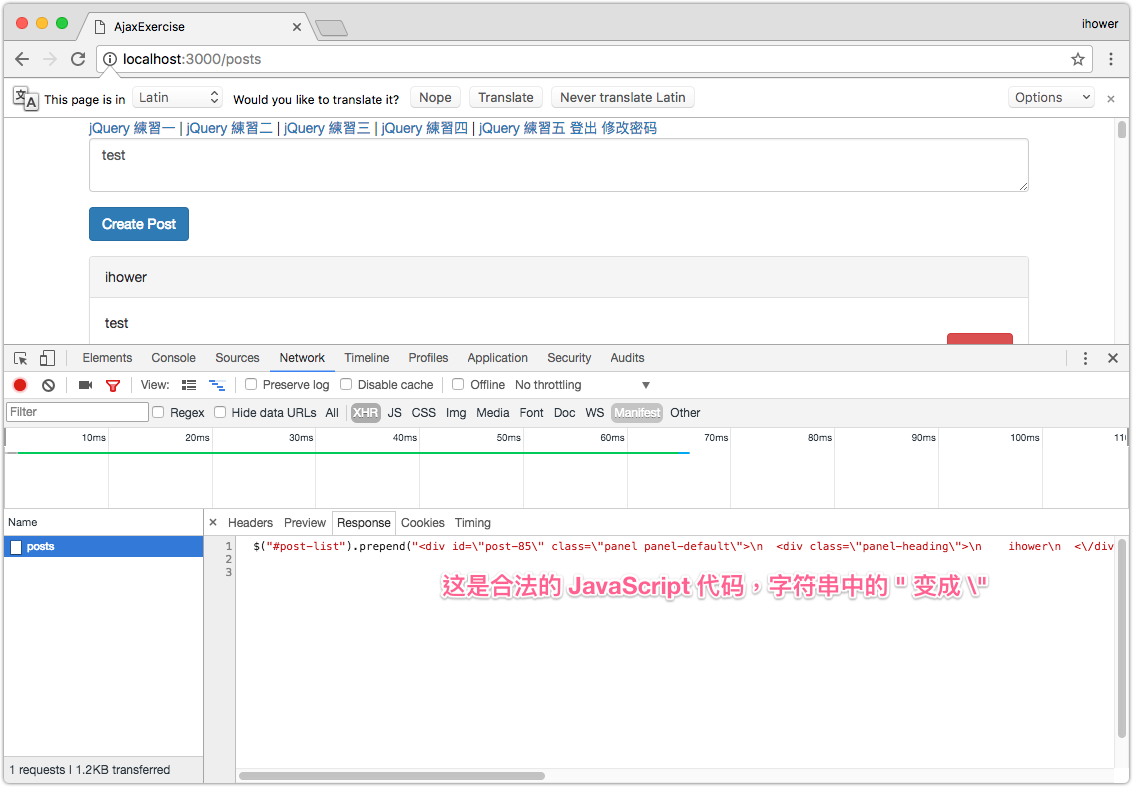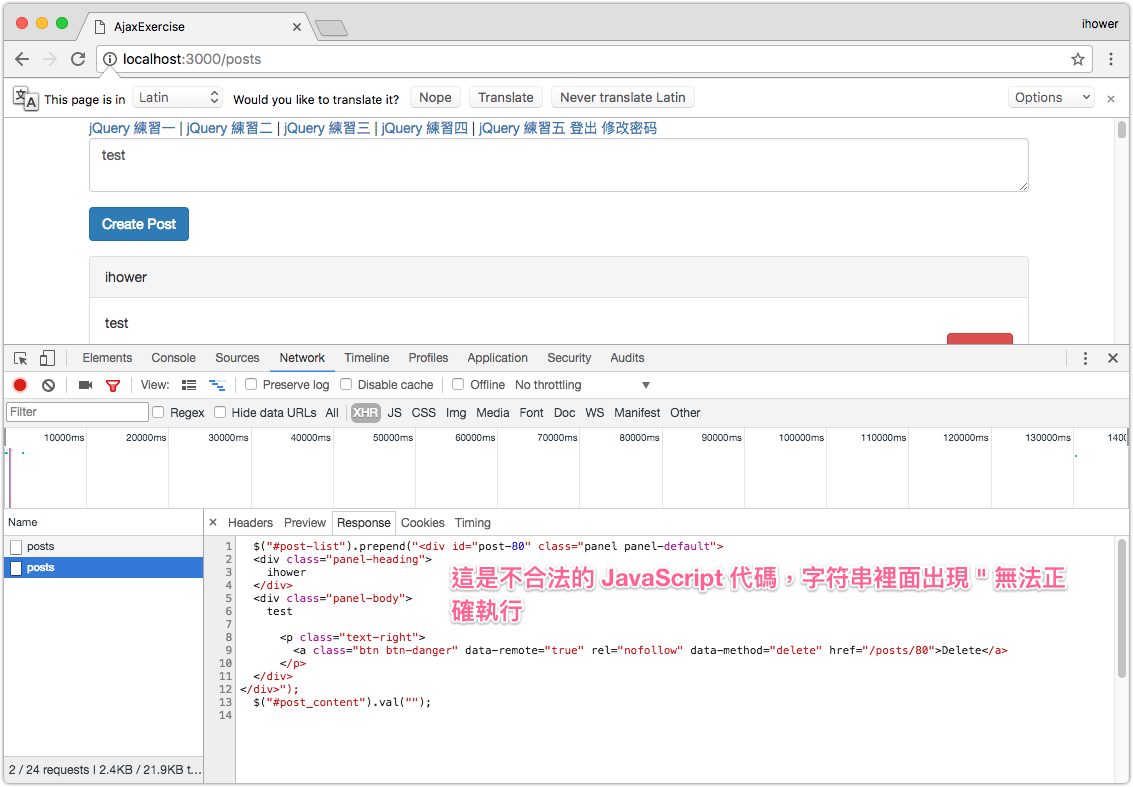## 2-8 Ajax 新增: 清空表單和錯誤處理

``````   \$("#post-list").prepend("<%=j render :partial => "post", :locals => { :post => @post } %>");
+  \$("#post_content").val("");

``````

`.val("")` 就可以把輸入框的值設定為空。

``````+ <% if @post.valid? %>
\$("#post-list").prepend("<%=j render :partial => "post", :locals => { :post => @post } %>");
\$("#post_content").val("");
+ <% else %>
+ <% end %>

``````

## 2-9 製作按讚功能

``````  class CreateLikes < ActiveRecord::Migration[5.0]
def change
create_table :likes do |t|
+       t.integer :user_id, :index => true
+       t.integer :post_id, :index => true
t.timestamps
end
end
end

``````

``````+  belongs_to :user
+  belongs_to :post

``````

``````   belongs_to :user

+  has_many :likes, :dependent => :destroy
+  has_many :liked_users, :through => :likes, :source => :user

+  def find_like(user)
+    self.likes.where( :user_id => user.id ).first
+  end

``````

``````
has_many :posts

+  has_many :likes, :dependent => :destroy
+  has_many :liked_posts, :through => :likes, :source => :post

``````

``````-  resources :posts
+  resources :posts do
+    member do
+      post "like" => "posts#like"
+      post "unlike" => "posts#unlike"
+    end
+  end

``````

``````  <div class="panel-body">
<%= post.content %>

+    <div class="text-right">
+
+      <% if post.liked_users.any? %>
+        <%= post.liked_users.map{ |u| u.display_name }.join(",") %> 點了贊
+      <% end %>
+
+      <% if current_user # 有登入才可以按讚 %>
+        <% if post.find_like(current_user) %>
+          <%= link_to "-1", unlike_post_path(post), :method => :post, :class => "btn btn-primary" %>
+        <% else %>
+          <%= link_to "+1", like_post_path(post), :method => :post, :class => "btn btn-primary" %>
+        <% end %>
+      <% end %>

# (略，刪除按鈕在這裡)

+    </div>
</div>

``````

``````
+  def like
+    @post = Post.find(params[:id])
+    unless @post.find_like(current_user)  # 如果已經按讚過了，就略過不再新增
+      Like.create( :user => current_user, :post => @post)
+    end
+
+    redirect_to posts_path
+  end
+
+  def unlike
+    @post = Post.find(params[:id])
+    like = @post.find_like(current_user)
+    like.destroy
+
+    redirect_to posts_path
+  end
+
``````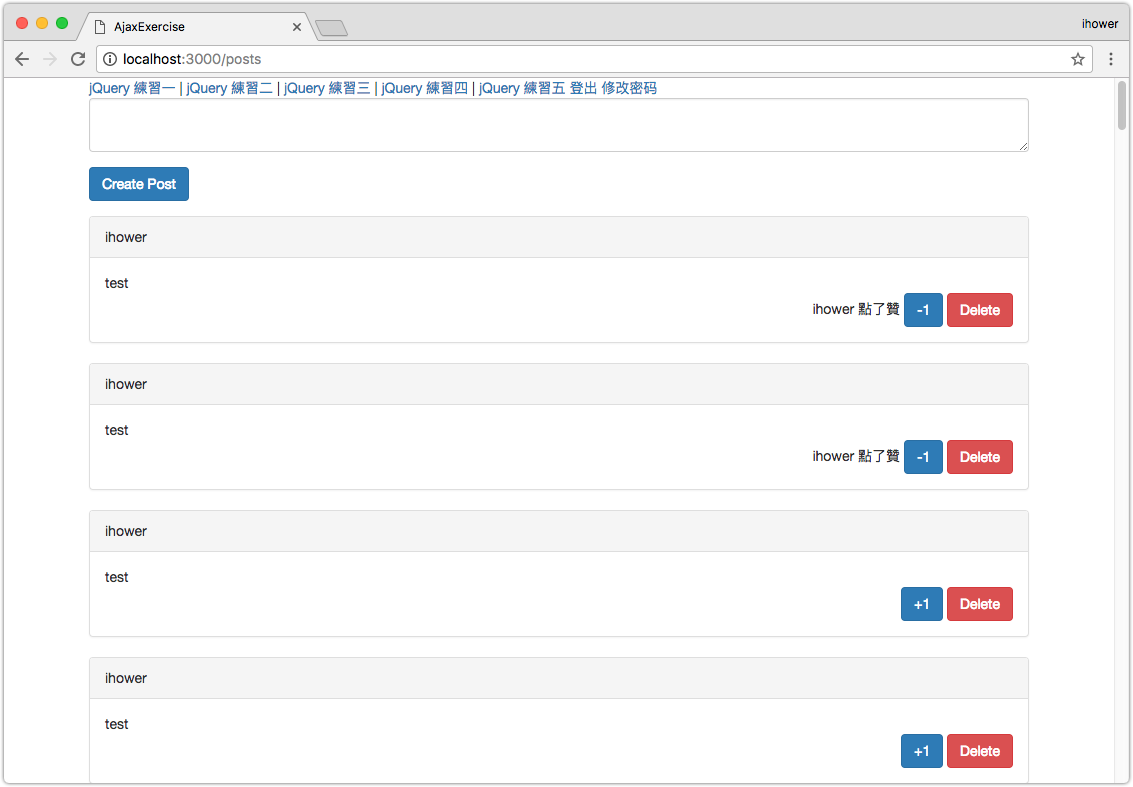## 2-10 Ajax 按讚

1. 把本來的 +1 和 -1 按鈕，改成 `:remote => true` 用 Ajax 送出
2. 伺服器會新建或刪除 Like 資料進資料庫
3. 伺服器回傳的 JavaScript 要替換掉整塊按讚的 HTML 部分，包括 XXX 按了讚，以及把 +1 改成 -1 按鈕或 -1 改成 +1 按鈕。

``````
-   <% if post.liked_users.any? %>
-     <%= post.liked_users.map{ |u| u.display_name }.join(",") %> 點了贊
-   <% end %>
-
-   <% if current_user # 有登入才可以按讚 %>
-     <% if post.find_like(current_user) %>
-       <%= link_to "-1", unlike_post_path(post), :method => :post, :class => "btn btn-primary" %>
-     <% else %>
-       <%= link_to "+1", like_post_path(post), :method => :post, :class => "btn btn-primary" %>
-     <% end %>
-   <% end %>

+    <span id="post-like-<%= post.id %>">
+      <%= render :partial => "like", :locals => { :post => post } %>
+    </span>

``````

``````<% if post.liked_users.any? %>
<%= post.liked_users.map{ |u| u.display_name }.join(",") %> 點了贊
<% end %>

<% if current_user # 有登入才可以按讚 %>
<% if post.find_like(current_user) %>
<%= link_to "取消讚", unlike_post_path(post), :method => :post, :remote => true, :class => "btn btn-primary" %>
<% else %>
<%= link_to "讚", like_post_path(post), :method => :post, :remote => true, :class => "btn btn-primary" %>
<% end %>
<% end %>

``````

``````
def like
# (略)
-   redirect_to posts_path
end

def unlike
# (略)
-   redirect_to posts_path
+   render "like"
end

``````

``````str = "<%=j render :partial => "like", :locals => { :post => @post } %>";
\$("#post-like-<%= @post.id %>").html(str);

``````

## 2-11 顯示和更新多少人按讚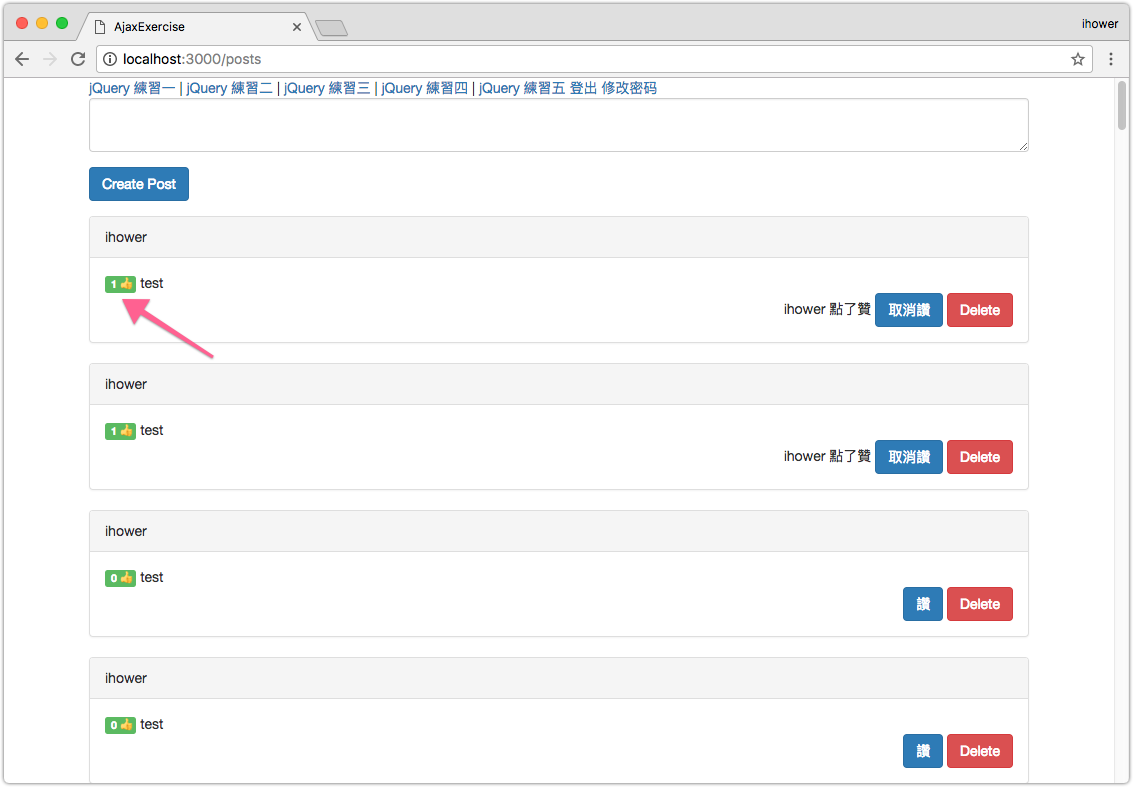``````   <div class="panel-body">
+    <span id="post-thumbsup-<%= post.id %>" class="label label-success"><%= post.likes.count %> 👍</span>

``````

``````   str = "<%=j render :partial => "like", :locals => { :post => @post } %>";
\$("#post-like-<%= @post.id %>").html(str);

+  \$("#post-thumbsup-<%= @post.id %>").html("<%= @post.likes.count %>" + " 👍")

``````

## 2-12 Ajax 小結

Ajax 是一種增強 UI 的方式，我們會先把基本功能完成，再根據需要改成 Ajax 效果。

Rails 內建透過 `:remote => true` 的方式，就可以很快製作出 Ajax 效果。 再稍後的章節中，我們會示範進階的用法：在一些情況下，我們必須手寫 jQuery 去綁事件送 Ajax，取得 JSON 回傳並更新 DOM。